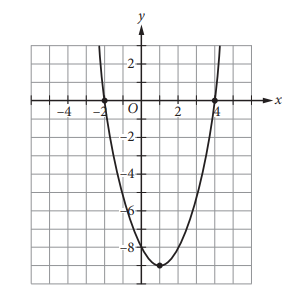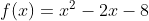Scan QR code or get instant email to install app

Question:

# The graph of y = f(x) is shown in this xy-plane. Which of the following equations could define f(x)?A 1212explanation

1212The graph of y = f(x) crosses the x-axis at x = −2 and x = 4, crosses the y-axis at y = 8, and has its vertex at the point (1, −9). Therefore, the ordered pairs (−2, 0), (4 0) , , (0, −8), and (1, −9) must satisfy the equation for f(x). Furthermore, because the graph opens upward, the equation defining f(x) must have a positive leading coefficient. All of these conditions are met by the equation.